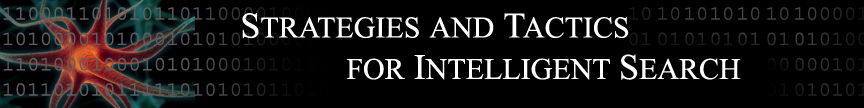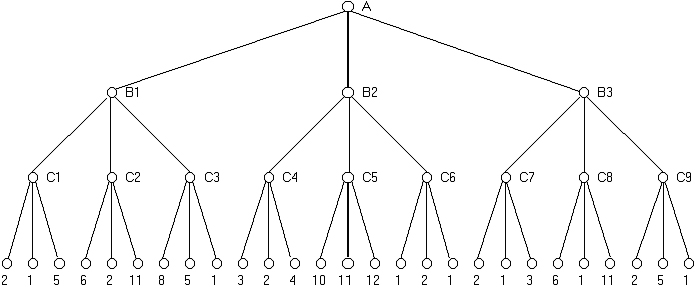ALGORITHMS - MINIMAX

Search algorithms tend to utilize a cause-and-effect concept--the search considers each possible action available to it at a given moment; it then considers its subsequent moves from each of those states, and so on, in an attempt to find terminal states which satisfy the goal conditions it was given. Upon finding a goal state, it then follows the steps it knows are necessary to achieve that state.

However, in a competitive multi-player game, when other agents are involved and have different goals in mind (and agents usually in fact have goals which directly oppose each other), things get more complicated. Even if a search algorithm can find a goal state, it usually cannot simply take a set of actions which will reach that state, since for every action our algorithm takes towards its goal, the opposing player can take an action which will alter the current state (presumably in a way unfavorable to our algorithm.) This does not mean searches are useless in finding strategies for multi-player games; they simply require additional tactics to be effective.

For two player games, the minimax algorithm is such a tactic, which uses the fact that the two players are working towards opposite goals to make predictions about which future states will be reached as the game progresses, and then proceeds accordingly to optimize its chance of victory. The theory behind minimax is that the algorithm's opponent will be trying to minimize whatever value the algorithm is trying to maximize (hence, "minimax"). Thus, the computer should make the move which leaves its opponent capable of doing the least damage.

In the ideal case (i.e., the computer has infinite time and infinite storage capacity), the computer will investigate every game outcome possible from the game's current state (as well as the paths taken to reach those states) and assign those outcomes values. For a win-or-lose game like chess or tic-tac-toe, there are only three possible values-win, lose, or draw (often assigned numeric values of 1, -1, and 0 respectively)--but for other games like backgammon or poker with a "score" to maximize, the scores themselves are used. Then, starting from the bottom, the computer evaluates which possible outcome is best for the computer's opponent. It then assumes that, if that game stage is reached, its opponent will make the move which leads to the outcome best for the opponent (and worse for the computer). Thus, it can "know" what its opponent will do, and have a concrete idea of what the final game state will be if that second-to-last position is in fact reached. Then, the computer can treat that position of a terminal node of that value, even though it is not actually a terminal value. This process can then be repeated a level higher, and so on. Ultimately, each option that the computer currently has available can be a assigned a value, as if it were a terminal state, and the computer simply picks the highest value and takes that action. A relatively simple example of this is shown below: